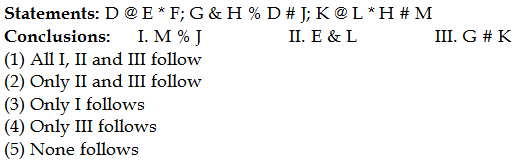## PREPARATORY QUESTIONS

### EQUAL-INEQUAL

#### PREPARATORY SET-27 (HARD)

Direction (Qs.1 to 5): In the following questions, the symbols #, %, &, @ and * are used with the following meanings as illustrated below, answer the questions accordingly.

‘A # B’ means ‘A is not greater than B’

‘A % B’ means ‘A is neither greater than nor equal to B’

‘A & B’ means ‘A is neither greater than nor less than B’

‘A @ B’ means ‘A is neither less than nor equal to B’

‘A * B’ means ‘A is not less than B’

Now in each of the following questions assuming the given statement to be true, find which of the conclusions given below them is/are definitely true and give your answer accordingly.

Question No : 1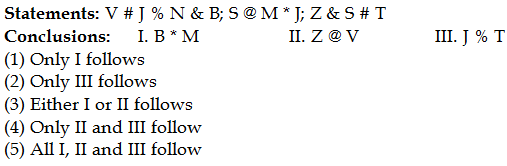Question No : 2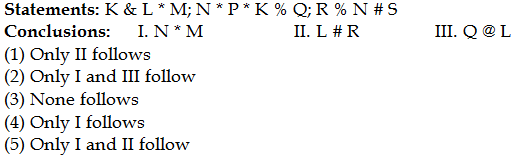Question No : 3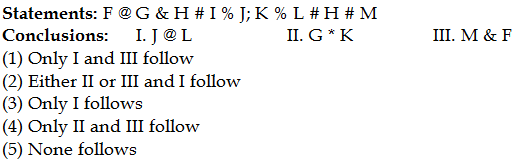Question No : 4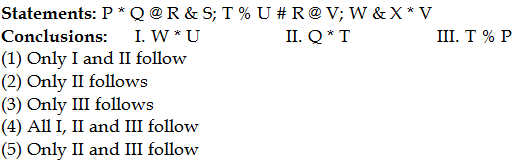Question No : 5